# pandas DataFrames¶

xlSlim was designed to support pandas DataFrames efficiently while keeping the interaction with Excel as intuitive as possible.

Note

Type hints are essential for xlSlim to recognise pandas DataFrames passed between your functions.

Warning

pandas functionality requires a premium licence. See Licensing

## Creating pandas DataFrames¶

pandas DataFrames can be created in three ways:
• within a Python function
• from an Excel range
• from an Excel range of numbers (optimised)

### pandas DataFrames from a Python function¶

This Python code defines a function create_random_df() that returns a DataFrame of random numbers between 0 and the number of rows, the index is a DataFrame of integers for to the number of rows.

```import pandas as pd
import numpy as np
from typing import List, Optional

def create_random_df(
minimum: Optional[int] = 0,
maximum: Optional[int] = 100,
rows: Optional[int] = 1,
columns: Optional[int] = 1,
) -> pd.DataFrame:
"""Create a DataFrame of random integers."""
return pd.DataFrame(
np.random.randint(minimum, maximum, size=(rows, columns)),
columns=[f"Col{i}" for i in range(columns)],
)
```

Note

All the Python code and Excel files shown are available from github in the xlslim-code-samples repo. I highly recommend downloading the samples from github. The Excel workbooks contain many tips and tricks.

Save the Python code as a new file on your PC. I saved the file in my Documents folder.

Open Excel and enter this `RegisterPyModule()` formula (amending the location to match where you saved the file):

```=RegisterPyModule("c:\users\russe\documents\DataFrame.py","C:\Users\russe\anaconda3\envs\py37")
```

Note

pandas and numpy are not available in the Python installation bundled with xlSlim. The Python module must be registered with an existing Python installation that has numpy and pandas installed.

If we call the create_random_df() function we see that the result is automatically cached and a cache handle is returned to Excel.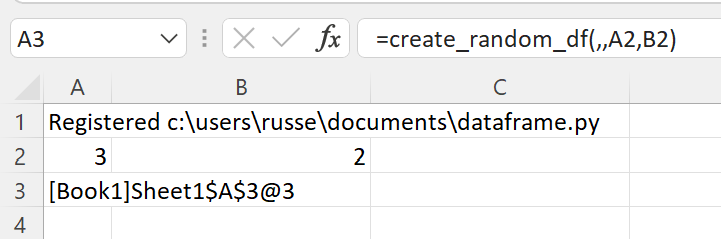The xlSlim function `ViewPyObject()` can be used to view any Python object stored in the memory cache. We see the DataFrame has three rows and two columns as expected.

```=ViewPyObject(A3)
```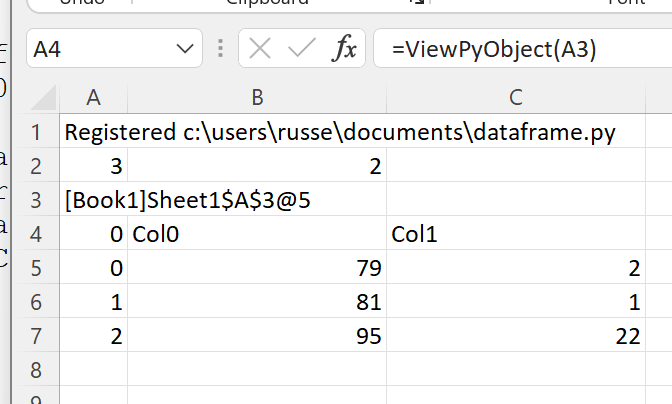### pandas DataFrame from an Excel Range¶

Any Excel range can be used to create a pandas DataFrame, however xlSlim does make assumptions about the columns and index. The first column is assumed to be the index. The first row is assumed to contain the column names. The top left cell is ignored. This does prevent the use of advanced indexing, DataFrames with advanced indexing should be created within Python functions as shown above.

Add this describe_df() function to the Python code and re-register the module with `RegisterPyModule()`

```def describe_df(x: pd.DataFrame) -> pd.DataFrame:
"""Describe this DataFrame."""
return x.describe()
```

This function describes the pandas DataFrame passed in, we’ll use it to show how xlSlim creates pandas DataFrame directly from Excel if the Python function has a type hint for pd.DataFrame.

We create a table of strings and numbers in Excel and pass this to the describe_df() function.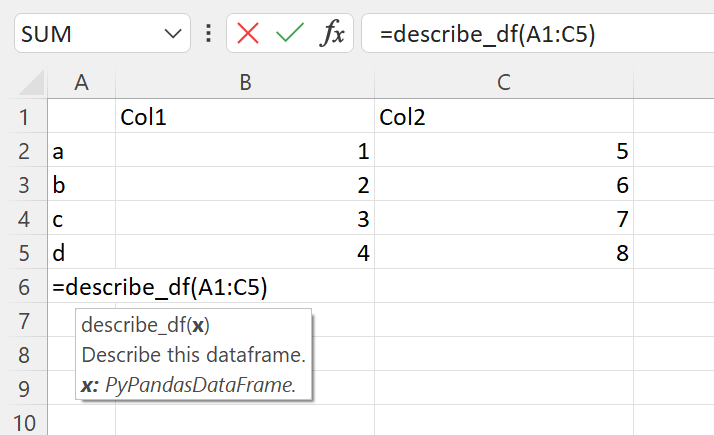The function returns a cached object handle to Excel.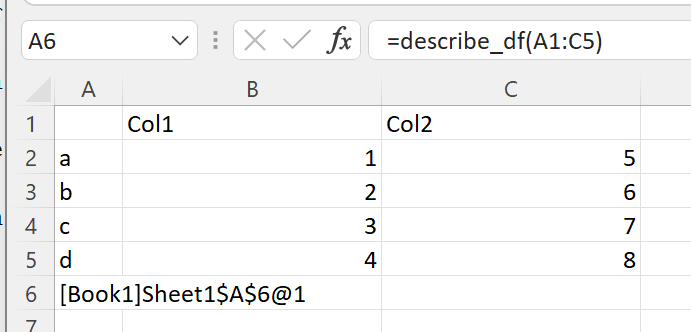A few things have happened here. xlSlim created a pandas DataFrame from the range A1:C5 before passing the data to the Python code. The Python code received a regular pandas DataFrame as expected. The Python function describe_df() then returned a new pandas DataFrame. xlSlim automatically put this pandas DataFrame into a memory cache and returned the cache handle to Excel.

Using `ViewPyObject()` we can see the DataFrame has been created and passed around as expected.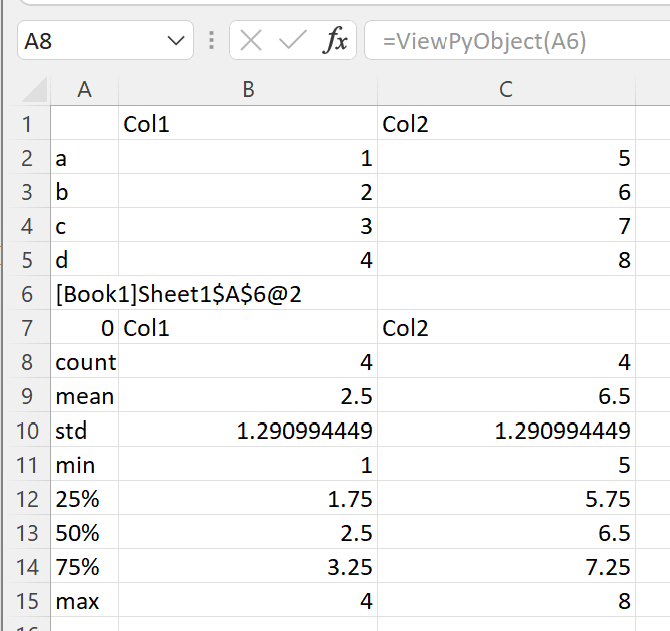### pandas DataFrame from an Excel Range of numbers¶

xlSlim is optimised to create pandas DataFrames from Excel ranges of numbers. Ranges of numbers are converted to pandas DataFrames very efficiently with only direct memory copies being used.

xlSlim cannot determine if the Excel range passed in only contains numbers (Technically it could but this would be very slow). Therefore xlSlim creates a special version of every Python function that expects one or more pandas DataFrames - these special functions have the same names with “_num_only” appended.

Let’s create a range of numbers and try the describe_df_num_only() function.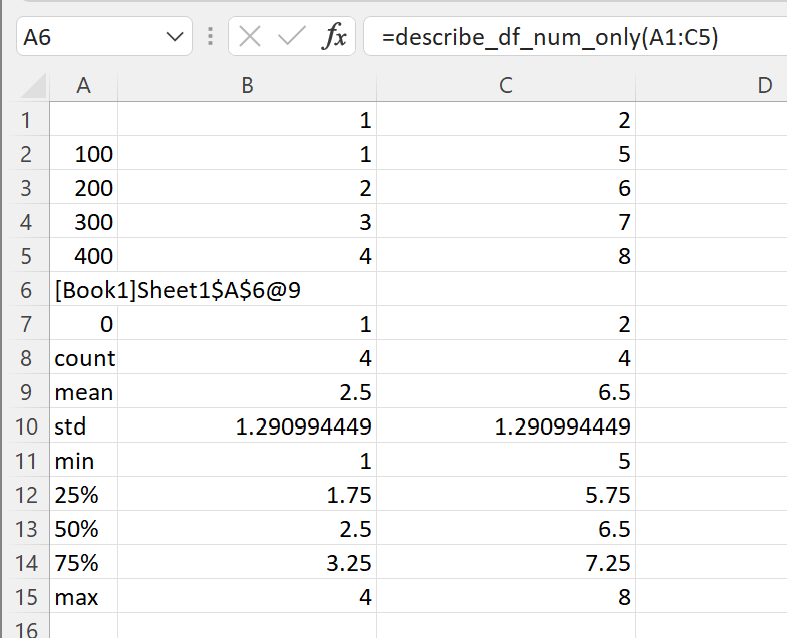The function describe_df_num_only() behaves exactly the same as describe_df() however it is far more efficient when passing in a range of numbers.

Note

For optimal performance always use the “_num_only” versions of xlSlim functions when creating numeric pandas DataFrames from Excel Ranges.

## Passing pandas DataFrames to Python functions¶

As shown above, pandas DataFrames can be created from Excel Ranges. xlSlim will create a pandas DataFrame from an Excel Range before passing the pandas DataFrame to the Python function.

Another way to pass pandas DataFrames is to use the cache object handles corresponding to pandas DataFrames in xlSlim’s memory cache.

Let’s create a DataFrame using the create_random_df() function we defined before.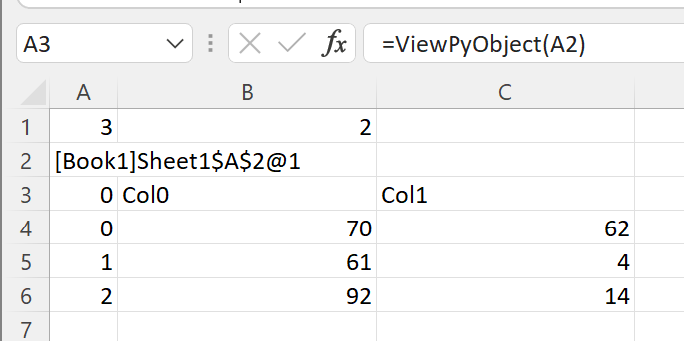The `ViewPyObject()` in A3 shows the DataFrame.

Remove the `ViewPyObject()` function and replace it with the describe_df_from_handle() function

```=describe_df_from_handle(A2)
```

This takes in the cache object handle from A2, gets the associated pandas DataFrame from xlSlim’s memory cache, passes the pandas DataFrame to the describe_df() Python function, and finally stores the result in the memory cache passing a cache object handle back to Excel.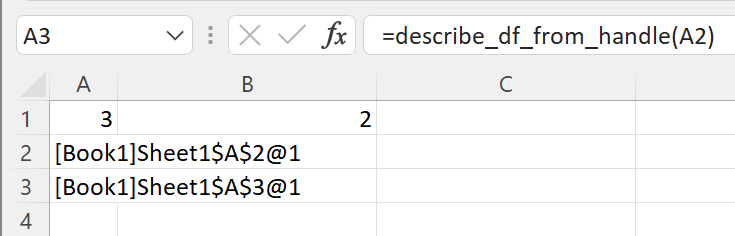The pandas DataFrame describing the object referred to in A2 can be viewed with `ViewPyObject()` as usual.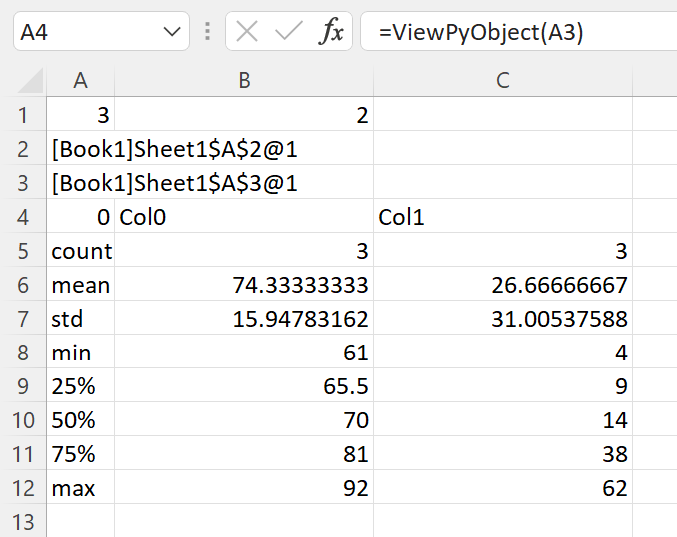Note

Passing pandas DataFrame using cache object handles is much more efficient than creating pandas DataFrame from Excel Ranges.

## Passing multiple pandas DataFrames to Python functions¶

Multiple pandas DataFrames can be passed to Python functions in two ways:
• As separate arguments as shown in the concat_two_df() function below
• As a list of numpy arrays as shown in the concat_df() function below
```from typing import List
XLSLIM_INWINDOWFUNC = None

def concat_two_df(
ds_a: pd.DataFrame,
ds_b: pd.DataFrame,
axis: Optional[int] = 0,
join: Optional[str] = "outer",
ignore_index: Optional[bool] = False,
) -> pd.DataFrame:
"""Concatenate the two supplied DataFrames."""
return concat_df([df_a, df_b], axis, join, ignore_index)

def concat_df(
series: List[pd.DataFrame],
axis: Optional[int] = 0,
join: Optional[str] = "outer",
ignore_index: Optional[bool] = False,
) -> pd.DataFrame:
"""Concatenate the supplied DataFrames."""
# Note how this global function can be used to skip
# running slow code in the Excel function wizard
if XLSLIM_INWINDOWFUNC and XLSLIM_INWINDOWFUNC():
return pd.DataFrame()
return pd.concat(dframes, axis=axis, join=join, ignore_index=ignore_index)
```

Given this sheet, where cache object handles in A2 and D2 reference pandas DataFrames created from create_random_df() and `ViewPyObject()` is used to display the DataFrames at A3 and D3: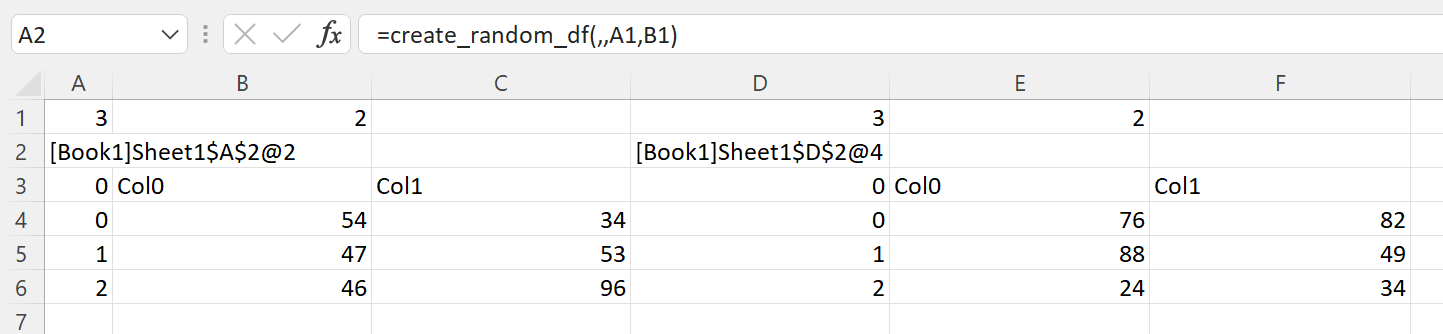For the first case, functions with separate arguments, two of the techniques discussed so far can be use. These are both valid

```=concat_two_df(A3#,D3#,,"outer")
=concat_two_df_from_handle(A2,D2,,"outer")
```

The column names are not numeric, so this fails. If the column names were numeric it would work.

```=concat_two_df_num_only(A3#,D3#,,"outer")
```

For the second case, functions expecting a list, only a list of cache object handles can be supplied.

```=concat_df(CreateRange(A2,D2),,"outer")
```

We use the `CreateRange()` utility function to create a range as A2 and D2 are not adjacent cells.

Note the use of `XLSLIM_INWINDOWFUNC()` to not concatenate DataFrames if the Excel function wizard is open.